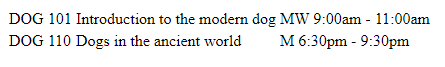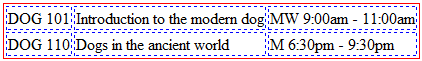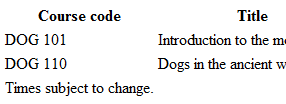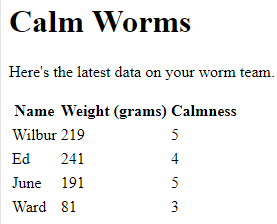# Tables

Tags

Lesson contents

### Basic tables

Tables are made up of rows and columns. Here's a table listing some university classes:It's hard to see the rows and columns, so let's add some lines.You can see that the table has two rows and three columns. That's what makes a table a table: rows and columns.

Here's the code for the table.

• <table>
•   <tbody>
•     <tr>
•       <td>DOG 101</td>
•       <td>Introduction to the modern dog</td>
•       <td>MW 9:00am - 11:00am</td>
•     </tr>
•     <tr>
•       <td>DOG 110</td>
•       <td>Dogs in the ancient world</td>
•       <td>M 6:30pm - 9:30pm</td>
•     </tr>
•   </tbody>
• </table>

The whole thing is surrounded by a `table` tag. Next is `thead`, giving table column headers. This table doesn't have any. After that is `tbody`, with the body of the table. Each row in the body is inside a `tr` tag. Inside each `tr`, there is a `td` for each table cell. The "d" in `td` stands for "data." There's one `td` for each column, in each row.

Here's the table of university classes again:Here's the code. The table is divided into three parts: the header, the body, and the footer.

• <table>
•     <tr>
•       <th>Course code</th>
•       <th>Title</th>
•       <th>When offered</th>
•     </tr>
•   <tbody> Body starts
•     <tr>
•       <td>DOG 101</td>
•       <td>Introduction to the modern dog</td>
•       <td>MW 9:00am - 11:00am</td>
•     </tr>
•     <tr>
•       <td>DOG 110</td>
•       <td>Dogs in the ancient world</td>
•       <td>M 6:30pm - 9:30pm</td>
•     </tr>
•   </tbody> Body ends
•   <tfoot> Footer starts
•     <tr>
•       <td colspan="3">Times subject to change.</td>
•     </tr>
•   </tfoot> Footer ends
• </table>

The header uses the tag `th` instead of `td`.

•   <tr>
•     <th>Course code</th>
•     <th>Title</th>
•     <th>When offered</th>
•   </tr>

By default, browsers render `th` as centered and bold. You can change that with CSS.

Here's the footer:

• <tfoot>
•   <tr>
•     <td colspan="3">Times subject to change.</td>
•   </tr>
• </tfoot>

The `colspan` tells the footer's `td` to take up three columns, so it stretches across the entire row as needed:Without the `colspan`...

• <tfoot>
•   <tr>
•     <td>Times subject to change.</td>
•   </tr>
• </tfoot>

... the table would look like this:The browser makes the first column too wide, so it can fit the text "Times subject to change."

Putting the `colspan` in the footer...

• <tfoot>
•   <tr>
•     <td colspan="3">Times subject to change.</td>
•   </tr>
• </tfoot>

... makes the table look more natural:### Exercise

Exercise

Calm worms

Use the `&lt;table&gt;` tag to make a page like this:Use any other tags you need.

• The `table` tag makes a table with rows and columns.
• A table can have a head (`thead`), a body (`tbody`), and a footer (`tfoot`).
• `tr` makes a table row.
• Inside `tr`s, `td` makes a table column.
• `colspan` makes a `td` span multiple columns.